Example Questions

Example Question #1 : How To Order Fractions From Least To Greatest Or From Greatest To Least

Order the following fractions from least to greatest:

2/3, 3/4, 1/2

3/4, 2/3, 1/2

2/3, 1/2, 3/4

1/2, 2/3, 3/4

2/3, 3/4, 1/2

3/4, 1/2, 2/3

1/2, 2/3, 3/4

Explanation:

1/2 is the least, then 2/3, then 3/4.

Example Question #2 : How To Order Fractions From Least To Greatest Or From Greatest To Least

Place the following fractions in order from greatest to least.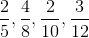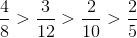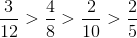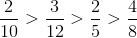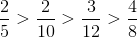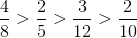Explanation:

This question can be most easily answered by converting the fractions into decimals first.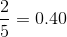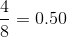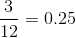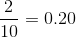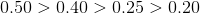Example Question #3 : How To Order Fractions From Least To Greatest Or From Greatest To Least

Order the following fractions from least to greatest: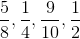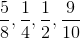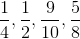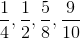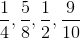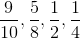Explanation:

The most generic process of ordering fractions that can apply to all questions begins with finding the common denominator between all fractions, preferably the least common denominator. In this question, the LCD is 40. Therefore the fractions become: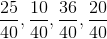. From this point, we can simply arrange the fractions based upon their numerators. This becomes: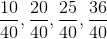. The final step is to reduce the fractions to their original denominators, which becomes:.

If you are comfortable and competent in quickly converting fractions to decimals, then there is a quicker method than what is described above. Simply convert each fraction to a decimal, organize the decimals, and then convert the decimals back to fractions. In this question we can convertto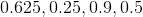. Then we can reorganize this to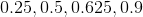and convert back to.

When approaching this kind of problem, choose the method in which you are more comfortable working with. There is no sense in trying to save time by choosing a method that you are not comfortable with and increasing your risk of answering the question incorrectly.

Example Question #4 : How To Order Fractions From Least To Greatest Or From Greatest To Least

Place the following fractions in increasing order: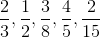.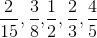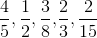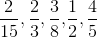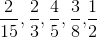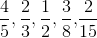To put these fractions in increasing order it is important to compare them as decimals, since fractions can often be deceiving.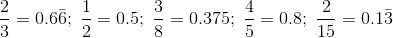.